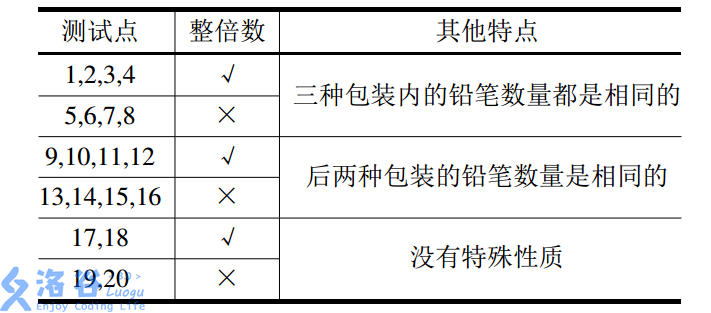P1422 小玉家的电费

### 题目描述

#### 输入输出样例

267

##### 输出

121.5

#include "stdio.h"
int main()
{
int a;
float b=0;
scanf("%d", &a);
if (a <= 150)
{
b = a * 0.4463;
}
if (a >= 151 && a <= 400)
{
b = 0.4463 * 150 + (a - 150) * 0.4663;
}
if(a>401)
{
b = 0.4463 * 150 + 250 * 0.4663 + (a - 400) * 0.5663;
}
printf("%.1f", b);
return 0;
}

P1085 不高兴的津津

### 题目描述

5 3
6 2
7 2
5 3
5 4
0 4
0 6

3

#### 说明/提示

noip2004普及组第1题

#include "stdio.h"
int main()
{
int a, b, c=0, i, max=0;
for (i = 0; i < 7; i++)
{
scanf("%d%d", &a[i], &b[i]);
if (a[i] + b[i] > 8 && a[i]+b[i]>max)
{
max = a[i] + b[i];
c = i+1;
}
}
printf("%d", c);
return 0;
}



P1089 津津的储蓄计划

### 题目描述

#### 输入格式

12行数据，每行包含一个小于350的非负整数，分别表示1月到12月津津的预算。

#### 输入输出样例

290
230
280
200
300
170
340
50
90
80
200
60

-7

290
230
280
200
300
170
330
50
90
80
200
60

##### 输出

1580

#include "stdio.h"
int main()
{
int hua, ziji = 0, h = 0;
for (int i = 1; i <= 12; i++)
{
scanf("%d", &hua);
ziji = ziji + 300 - hua;
if (ziji < 0)
{
printf("-%d", i);
return 0;
}
else
{
h = ziji / 100 + h;
ziji = ziji % 100;
}
}
printf("%d", 120 * h + ziji);
return 0;
}

P1909 买铅笔

### 题目描述

P老师需要去商店买n支铅笔作为小朋友们参加NOIP的礼物。她发现商店一共有3种包装的铅笔，不同包装内的铅笔数量有可能不同，价格也有可能不同。为了公平起 见，P老师决定只买同一种包装的铅笔。

#### 输出格式

11个整数，表示P老师最少需要花费的钱。

57
2 2
50 30
30 27

54

9998
128 233
128 2333
128 666

18407

9999
101 1111
1 9999
1111 9999

89991

2支装，价格为2;
50支装，价格为30;
30支装，价格为27。
P老师需要购买至少57支铅笔。

### 【子任务】#include<stdio.h>
int main() {
int n;
scanf("%d", &n);
int i;
int min = 0;
int a;
int b;
for (i = 0; i < 3; i++) {
scanf("%d%d", &a[i], &b[i]);
int N = 0;
int sum = 0;
while (1) {
N += a[i];
sum += b[i];
if (N >= n) {
break;
}
}
if (i == 0)    min = sum;
if (min > sum)    min = sum;
}
printf("%d", min);
return 0;
}